Ponderomotive squeezing and entanglement in a ring cavity with two vibrational mirrors*
Laboratory of Nanophotonic Functional Materials and Devices (SIPSE), Laboratory of Quantum Engineering and Quantum Materials, South China Normal University, Guangzhou 510006, China

Corresponding author. E-mail: zmzhang@scnu.edu.cn

*Project supported by the Major Research Plan of the National Natural Science Foundation of China (Grant No. 91121023), the National Natural Science Foundation of China (Grant Nos. 61378012 and 60978009), the Specialized Research Fund for the Doctoral Program of Higher Education of China (Grant No. 20124407110009), the National Basic Research Program of China (Grant Nos. 2011CBA00200 and 2013CB921804), and the Program for Changjiang Scholars and Innovative Research Team in University, China (Grant No. IRT1243).

Abstract

We investigate the properties of the ponderomotive squeezing and the entanglements in a ring cavity with two vibrational mirrors. In the part about squeezing, we find that the squeezing spectrum of the transmitted field shows a distinct feature when the two vibrational mirrors have different frequencies. We also study the effects of some external parameters such as the temperature and the laser power on the degree of squeezing. In the part concerning entanglement, we study the entanglements between the cavity field and one of the vibrational mirrors, and that between the two vibrational mirrors, with emphasis focusing on the robustness of entanglements with respect to the environment temperature.

Keyword: 03.65.Ta; 03.65.Ud; 42.50.Lc; optomechanical cavity; squeezing; entanglement
1. Introduction

The optomechanical system has attracted much attention because of its potential applications in high precision measurements and quantum information processing. In a typical optomechanical system, a movable mirror couples to the cavity field via the radiation pressure, [3, 4] and this coupling can lead to many remarkable effects, for example, the optomechanically induced transparency (OMIT), [5, 6] the quantum ground state cooling of the nanomechanical resonators, [7, 8] entanglement[9, 10] and squeezing.[11, 12]

Entanglement is one of the most striking properties of quantum mechanics and has become a significant resource for quantum information processing. The entanglement beyond the microscopic level has inspired long-standing interest. It is widely accepted that this kind of entanglement should exist if the decoherence induced by the environment can be sufficiently suppressed. So in recent years there has been considerable interest in studying entanglement in mesoscopic and even macroscopic systems, and cavity optomechanical systems have become important candidates for generating quantum entanglement at the macroscopic level.[9, 10] Besides the basic entanglement between the cavity field and the vibrational mirror,  there are also entanglement between the two vibrational mirrors.

The optical squeezing is intimately linked to quantum-limited displacement sensing,  and the optomechanical method of manipulating the quantum fluctuations has been named as the ponderomotive squeezing. Just like the interaction between electromagnetic radiation and atoms which has led to an interesting quantum feature of squeezing, many are devoted to achieving the ponderomotively squeezed light because of its applications in ultrahigh precision measurements.

The variations beyond the standard optomechanics are abundant, such as a semitransparent membrane within a standard Fabry– Pé rot cavity,  nanoelectromechanical systems formed by a microwave cavity capacitively coupled to a nanoresonator, [19, 20] and ultracold atoms interacting with an optical cavity.

In this paper, we study the squeezing spectrum of the transmitted field and the entanglements in a ring cavity with two vibrational mirrors. This system is similar to that proposed in Ref. . In such a system, the two vibration mirrors owning the same or different frequencies will present distinct features. One of the features is double-optomechanically induced transparency. Here we study the features of the squeezing spectrum and show how the degree of squeezing changes with the external parameters such as the temperature and the input laser power. As the entanglement between the two vibration mirrors has been demonstrated in Ref.  using the criterion proposed in Ref. , here we mainly study the degree and the robustness of entanglement with respect to the environment temperature.

The organization of this paper is as follows. In Section 2 we describe the model and present the equations of motion in the frequency domain and in the time domain. We study the ponderomotive squeezing in the frequency domain in Section 3, and the entanglements in the time domain in Section 4, respectively. Section 5 presents the conclusions.

2. Model and equations of motion

The system we considered is shown in Fig. 1, in which a single mode optical ring cavity consists of two vibrational perfectly reflecting mirrors (M1 and M2) and two fixed mirrors (F1 and F2), one of the fixed mirrors (labeled as F1) is a partially transmitting mirror and the other (labeled as F2) is a perfectly reflecting mirror. The vibrational mirrors M1 and M2 are treated as quantum mechanical oscillators with the same effective mass m, and their frequencies are ω m1 and ω m2, respectively. When a classical laser beam with frequency ω 0 is injected into the cavity through the partially transmitting mirror F1, the Hamiltonian of this system can be described as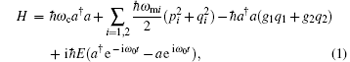where the first term is the Hamiltonian of the cavity field, whose annihilation and creation operators are a and a, respectively, and ω c is the resonant frequency of the cavity in the absence of the injected laser beam. The second term is the Hamiltonian of the vibrational mirrors with dimensionless position operators qi and momentum operators pi (i = 1, 2). The third term describes the nonlinear coupling of the vibrational mirrors to the cavity field via radiation pressure, where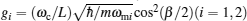is the coupling rate, with β the angle between the incident light and the reflected light at the surfaces of the movable mirrors and L the cavity length in the absence of the intracavity field. The last term describes the interaction of the cavity field with the pump field, with E the amplitude related to the input laser power P by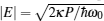, here κ is the cavity decay rate.

 Figure Option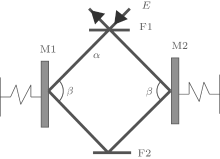Fig. 1. A ring cavity with four mirrors. A laser beam enters into the ring cavity through the fixed partially transmitting mirror F1. The fixed mirror F2 and the two vibrational mirrors (M1 and M2) are perfectly reflecting.

A proper analysis of the system must include photon losses in the cavity and the Brownian noise acting on the mirrors. This can be accomplished by considering the following set of nonlinear Langevin equations, written in the interaction picture with respect to ω 0: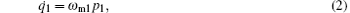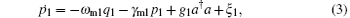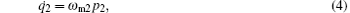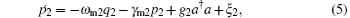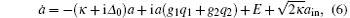where Δ 0 = ω cω 0, and γ mi (i = 1, 2) is the mechanical damping rate. ain is the input vacuum noise operator and its nonzero correlation function is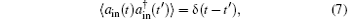and ξ is the thermal Langevin force, resulting from the coupling of vibrational mirrors to the environment, with correlation function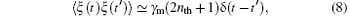where nth = [exp(ħ ω m/kBT) – 1]− 1 is the mean number of thermal excitation with kB being the Boltzmann constant.

From Eqs. (2)– (6) we can obtain the following steady-state mean-values: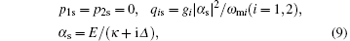where Δ is the effective cavity detuning given by Δ = Δ 0 − (g1q1s + g2q2s).

We can write each Heisenberg operator as a sum of its steady-state mean-value and an additional fluctuation operator with zero mean-value, i.e., a = α s + δ a, qi = qis + δ qi, p = pis + δ pi (i = 1, 2). When we substitute these expressions into Eqs. (2)– (6), and take into account the fact that for generating optomechanical entanglement the input laser power P is usually very large, this means | α s| ≫ 1, then we can neglect some small quantities and obtain following linearized Langevin equations: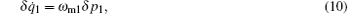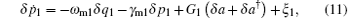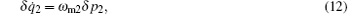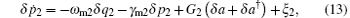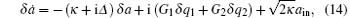where Gi = giα s(i = 1, 2) is the effective coupling strength.

Sometimes, it is more convenient to work in the frequency domain because fluctuations of the electric field are more convenient to be measured in the frequency domain than in the time domain. For calculating in frequency domain, we introduce the Fourier transform for an operator u (u = δ a, δ pi, δ qi, ain, and ξ i) as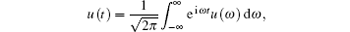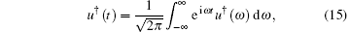in which [u(ω )] = u(− ω ). Then we rewrite Eqs. (10)– (14) in the frequency domain, which can be written in matrix form as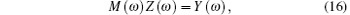where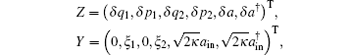and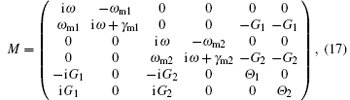in which we have defined Θ 1 = κ + i(Δ + ω ) and Θ 2 = κ + i(− Δ + ω ). By solving Eq. (16), we obtain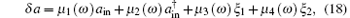where the coefficients are given as follows: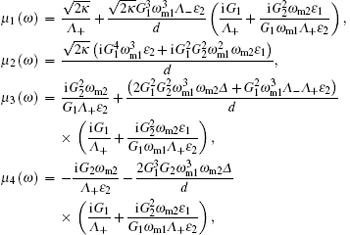in which we have defined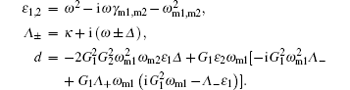In the following two sections we will study, respectively, the ponderomotive squeezing in the frequency domain, and the entanglements in the time domain.

3. Ponderomotive squeezing

We first analyze the squeezing properties of the transmitted field, which is accessible to experiment and useful for practical applications. The squeezing spectrum of the transmitted field is given by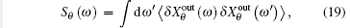where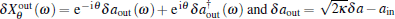. Here, in the input-output relation, the δ aout is used to describe the output quantum field which is the squeezed light in this system and the ain is the vacuum noise instead of the input laser. Therefore,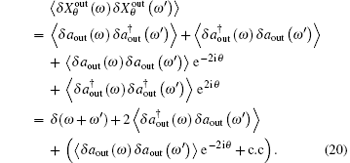By using the results of Eq. (18) and the following nonzero correlation functions in the frequency domain:[9, 26]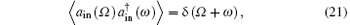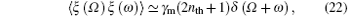we can further write Eq. (20) to be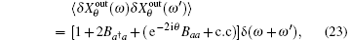in which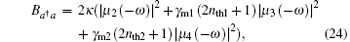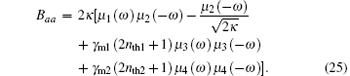Substituting Eq. (23) into Eq. (19), we can obtain the squeezing spectrum in a simple form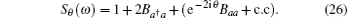As the phase angle θ is an adjustable parameter, we choose appropriate θ by solving dSθ (ω )/(dθ ) = 0, then we obtain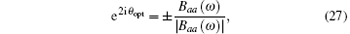here we choose the minus sign to optimize the degree of squeezing. Then the optimal squeezing spectrum can be written as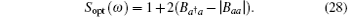In order to obtain squeezing, the term (Baa − | Baa| ) in the right-hand side must be negative,  i.e., Sopt(ω ) < 1. To illustrate the numerical results, we use the following set of experimentally realizable parameters throughout the paper:[29, 30]λ = 2π c/ω 0 = 1064 nm, ω m = 2π × 107 Hz, κ = π × 107 Hz, γ m1 = γ m2 = π × 400 Hz, L = 6 mm, m = 12 ng and we set the detuning and the angle as Δ 0 = 2π × 107 Hz and β = π /3, respectively. Then we show how the squeezing spectrum changes with respect to the external parameters.

Figure 2(a) shows that when the frequencies of the two vibrational mirrors are the same, the squeezing spectrum presents one peak at the frequencies ω = ω m which is similar to the spectrum of a typical optomechanical cavity. However, once we set the frequencies of the two vibrational mirrors with a difference, the original one peak splits into two peaks at the frequencies ω = ω m1 and ω m2 as shown in Fig. 2(b). This frequency difference also leads to a split in the optomechanically induced transparency window, as reported in Ref. .

 Figure Option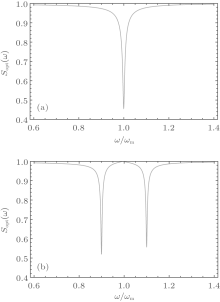Fig. 2. The squeezing spectrum with (a) ω m1 = ω m2 = ω m; (b) ω m1 = ω m + 0.1 × 2π × 107 Hz, ω m2 = ω m− 0.1 × 2π × 107 Hz. The other parameters are P = 6 mW, T1 = T2 = 3 mK, λ = 2π c/ω 0 = 1064 nm, ω m = 2π × 107 Hz, κ = π × 107 Hz, γ m1 = γ m2 = π × 400 Hz, L = 6 mm, m = 12 ng and we set the detuning and the angle as Δ 0 = 2π × 107 Hz and β = π /3, respectively.

From the expressions of the squeezing spectrum, it is easy to find that the degree of squeezing is related to the mean thermal phonon number nthi(i = 1, 2), which means that the environment temperature has important effects, and we show how the temperatures change the degree of the squeezing in such a system in Fig. 3. In Fig. 3(a), compared with Fig. 2(b), if we increase the temperature of mirror 1 from 3 mK to 10 mK and keep the temperature of mirror 2 at 3 mK, we find that the degree of squeezing at frequency ω = ω m1 decreases while there is no effect on the frequency ω = ω m2. In Fig. 3(b), when we increase the temperature of mirror 1 to 20 mK and still do not change the temperature of mirror 2, the frequency at ω = ω m1 will present no squeezing. Then we change the temperature of mirror 2 and keep the temperature of mirror 1 at 20 mK, and show the squeezing spectrum in Figs. 3(c) and 3(d). In this case, only the degree of squeezing at frequency ω = ω m2 changes. We can discuss the above phenomena physically as follows. As the noise reduction is a result of mechanical motion,  there is no squeezing when the coupling gi(i = 1, 2) is zero. Once the squeezing emerges, it occurs at the frequency corresponding to the mechanical frequency which we have shown in Figs. 2 and 3. In other words, each vibrational mirror has a direct effect on the degree of squeezing related to its own frequency.

 Figure Option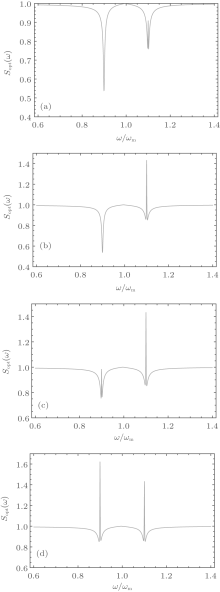Fig. 3. The squeezing spectrum with (a) T1 = 10 mK, T2 = 3 mK; (b) T1 = 20 mK, T2 = 3 mK; (c) T1 = 20 mK, T2 = 10 mK; (d) T1 = 20 mK, T2 = 20 mK. The other parameters are P = 6 mW, λ = 2π c/ω 0 = 1064 nm, ω m = 2π × 107 Hz, κ = π × 107 Hz, γ m1 = γ m2 = π × 400 Hz, L = 6 mm, m = 12 ng and we set the detuning and the angle are Δ 0 = 2π × 107 Hz and β = π /3, respectively.

At last, we show the effects of the power of laser on the degree of squeezing in Fig. 4. To clearly see these effects, we set the temperature of mirrors at T1 = T2 = 15 mK. In Figs. 4(a) and 4(b), we can find that as we increase the power of the laser, the degree of squeezing at both frequencies is enhanced. This is because the laser interacts with the two vibrational mirrors at the same time and a larger power will input more photons, which will effectively overcome the effects of thermal noise.

 Figure Option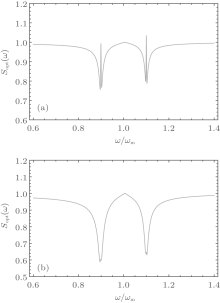Fig. 4. The squeezing spectrum with (a) P = 8 mW; (b) P = 20 mW. The other parameters are T1 = T2 = 15 mK, λ = 2π c/ω 0 = 1064 nm, ω m = 2π × 107 Hz, κ = π × 107 Hz, γ m1 = γ m2 = π × 400 Hz, L = 6 mm, m = 12 ng and we set the detuning and the angle as Δ 0 = 2π × 107 Hz and β = π /3, respectively.

4. Entanglements

In this section we discuss the entanglement between one of the vibrational mirrors and the cavity field (the mirror– cavity entanglement), and the entanglement between the two vibrational mirrors (the mirror– mirror entanglement). We define the cavity field quadratures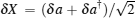and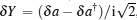, and the corresponding Hermitian input noise operators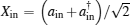and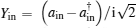, then equations (10)– (14) can be rewritten as the matrix form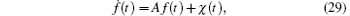in which the transposes of the column vectors f(t) and χ (t) can be expressed as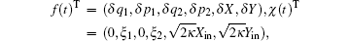respectively, and the matrix A is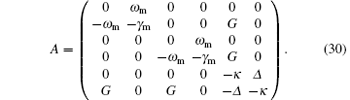Here, we have introduced the effective optomechanical coupling G = gα s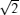, and assumed ω m1 = ω m2 = ω m, and γ m1 = γ m2 = γ m for simplicity. The solution of Eq. (29) is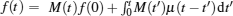, here M(t) = eAt. The system reaches its steady state as t → ∞ only if the real parts of all the eigenvalues of matrix A are negative so that M(∞ ) = 0. The stability conditions for the system can be found by employing the Routh– Hurwitz criterion. When the system is stable, it reaches a unique steady state. Owing to the quantum noises, ξ and ain are zero-mean quantum Gaussian noises and the dynamics is linearized, thus the quantum steady states for the fluctuations are a zero-mean tripartite Gaussian state, which is completely determined by the 6 × 6 correlation matrix Vij = 〈 fi(∞ )fj(∞ ) + fj(∞ )fi(∞ )〉 /2, here fT(∞ ) = (δ q1(∞ ), δ q2(∞ ), δ p1(∞ ), δ p2(∞ ), δ X(∞ ), δ Y(∞ )) is the vector of continuous variables fluctuations operators at the steady state (t → ∞ ). When the stability conditions are satisfied, the steady-state correlation matrix (CM) satisfies a Lyapunov equation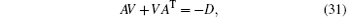where D = Diag[0, γ m(2th + 1), 0, γ m(2th + 1), κ , κ ] is a diagonal matrix and th is the mean thermal excitation number.

In order to investigate the behavior of the continuous variables (CV) entanglement between the elements of the tripartite system, we consider the logarithmic negativity Emc, a quantity which has already been proposed as a measure of entanglement,  to measure the entanglement between the cavity field and one of the mirrors (here we choose the left mirror of this system). We define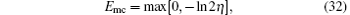where η = 2− 1/2{Σ (Γ ) − [Σ (Γ )2 − 4detΓ ]1/2}1/2 with Σ (Γ ) = detLm + detLf − 2detLmf, and we have used the 2 × 2 block form of the correlation matrix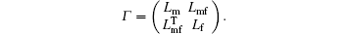Here, Γ is obtained by tracing out the mirror– mirror mode, i.e., removing the rows and columns of V corresponding to mirror– mirror. Lm, Lf, and Lmf are 2× 2 matrices, where Lm corresponds to the mechanical mode, Lf refer to the field mode and Lmf describes the correlation between these two modes. Similarly, we can quantify the bipartite entanglement of the mirror– mirror subsystem. To illustrate the numerical results, we choose the realistic parameters of the entanglement systems to be the same as in Section 3.

 Figure Option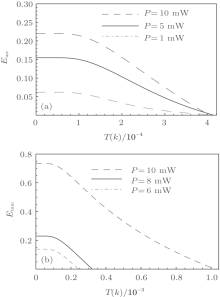Fig. 5. (a) Plot of the logarithmic negativity Emc versus the environment temperature. The red-dot-dashed, solid, blue-dashed curves refer to P = 1 mW, 5 mW, 10 mW, respectively. (b) Plot of the logarithmic negativity Emm versus the environment temperature. The red-dot-dashed, solid, blue-dashed curves refer to P = 6 mW, 8 mW, 10 mW, respectively. The other parameters are λ = 2π c/ω 0 = 1064 nm, ω m = 2π × 107 Hz, κ = π × 107 Hz, γ m1 = γ m2 = π × 400 Hz, L = 6 mm, m = 12 ng and we set the detuning and the angle are Δ 0 = 2π × 107 Hz, and β = π /3, respectively.

In Fig. 5(a) we plot the mirror– cavity entanglement Emc with respect to the environment temperature T. It can be seen that (i) Emc decreases with the increase of T; (ii) Emc increases with the increase of the laser power P; (iii) Emc disappears at almost the same temperature (about 0.4 mK) for different laser powers. The third point is an interesting phenomenon, which means there is nearly the same critical temperature for different powers. This is because when the temperature reaches the critical temperature, the effect of thermal noise on Emc is greater than that of increasing the laser power.

The mirror– mirror entanglement Emm as a function of the environment temperature is shown in Fig. 5(b). It can be seen that Emm has the features that are similar to the first two points of Emc. However, the robustness of Emm against T can be remarkably improved when we increase the laser power, this is very different from Emc. That is to say, for the mirror– mirror entanglement Emm, when the laser power is larger than 8 mW, it can effectively overcome the effect of thermal noise compared to Fig. 5(a).

5. Conclusion

In conclusion, we have studied the properties of the ponderomotive squeezing and the entanglements in a ring cavity with two vibrational mirrors. We have found that we can obtain a two-mode squeezed light in this system and can control the degree of squeezing flexibly by adjusting the related temperature or the laser power. We have also shown that the robustness of the mirror– mirror entanglement Emm with respect to the temperature is enhanced obviously when we increase the laser power. However, the mirror– cavity entanglement Emc has no such feature.

Reference
 1 Braginsky V B and Khalili F Y 1992 Quantum Measurement Cambridge Cambridge University Press [Cited within:2] 2 Nielson M A and Chuang I L 2000 Quantum Computation and Quantum Information Cambridge Cambridge University Press [Cited within:1] [JCR: 1.646] 3 Kippenberg T J and Vahala K J 2008 Science 321 1172 DOI:10.1126/science.1156032 [Cited within:1] 4 Marquardt F and Girvin S M 2009 Physics 2 40 DOI:10.1103/Physics.2.40 [Cited within:1] [CJCR: 0.2383] 5 Agarwal G S and Huang S 2010 Phys. Rev. A 81 041803 DOI:10.1103/PhysRevA.81.041803 [Cited within:1] [JCR: 3.042] 6 Weis S, Rivière R, Deléglise S, Gavartin E, Arcizet O, Schliesser A and Kippenberg T J 2010 Science 330 1520 DOI:10.1126/science.1195596 [Cited within:1] 7 Wilson-Rae I, Nooshi N, Zwerger W and Kippenberg T J 2007 Phys. Rev. Lett. 99 093901 DOI:10.1103/PhysRevLett.99.093901 [Cited within:1] [JCR: 7.943] 8 Liu Y C, Hu Y W, Wong C W and Xiao Y F 2013 Chin. Phys. B 22 114213 DOI:10.1088/1674-1056/22/11/114213 [Cited within:1] [JCR: 1.148] [CJCR: 1.2429] 9 Vitali D, Gigan S, Ferreira A, Böhm H R, Tombesi P, Guerreiro A, Vedral V, Zeilinger A and Aspelmeyer M 2007 Phys. Rev. Lett. 98http://dx.doi.org/10.1103/PhysRevLett.98.030405 030405 [Cited within:6] [JCR: 7.943] 10 Hartmann M J and Plenio M B 2008 Phys. Rev. Lett. 101 200503 DOI:10.1103/PhysRevLett.101.200503 [Cited within:2] [JCR: 7.943] 11 Clerk A A, Marquardt F and Jacobs K 2008 New J. Phys. 10 095010 DOI:10.1088/1367-2630/10/9/095010 [Cited within:1] [JCR: 4.063] 12 Fabre C, Pinard M, Bourzeix S, Heidmann A, Giacobino E and Reynaud S 1994 Phys. Rev. A 49 1337 DOI:10.1103/PhysRevA.49.1337 [Cited within:1] [JCR: 3.042] 13 Huang S M and Agarwal G S 2009 New J. Phys. 11 103044 DOI:10.1088/1367-2630/11/10/103044 [Cited within:4] [JCR: 4.063] 14 Braginsky V B and Manukin A B 1967 Sov. Phys. JETP 25 653http://www.jetp.ac.ru/cgi-bin/e/index/e/25/4/p653?a=list [Cited within:1] 15 Brooks D W C, Thierry B, Sydney S, Purdy T P, Nathan B and Stamper-Kurn D M 2012 Nature 488 476http://dx.doi.org/10.1038/nature11325 [Cited within:2] [JCR: 38.597] 16 Safavi-Naeini A H, Simon G, Hill J T, Jasper C, Markus A and Oskar P 2013 Nature 500 185 DOI:10.1038/nature12307 [Cited within:1] [JCR: 38.597] 17 Purdy T P, Yu P L, Peterson R W, Kampel N S and Regal C A 2013 Phys. Rev. X 3 031012http://dx.doi.org/10.1103/PhysRevX.3.031012 [Cited within:2] 18 Thompson J D, Zwickl B M, Jayich A M, Marquardt F, Girvin S M and Harris J G E 2008 Nature 452 72 DOI:10.1038/nature06715 [Cited within:1] [JCR: 38.597] 19 Teufel J D, Castellanos-Beltran M A, Harlow J W and Lehnert K W 2009 Nat. Nano. 4 820 DOI:10.1038/nnano.2009.343 [Cited within:1] 20 Ma P C, Xiao Y, Yu Y F and Zhang Z M 2014 Opt. Express 22 3621http://dx.doi.org/10.1364/OE.22.003621 [Cited within:1] [JCR: 3.546] 21 Brennecke F, Ritter S, Donner T and Esslinger T 2008 Science 322 235 DOI:10.1126/science.1163218 [Cited within:1] 22 Sun Q, Hu X, Ji A C and Liu W M 2011 Phys. Rev. A 83 043606 DOI:10.1103/PhysRevA.83.043606 [Cited within:1] [JCR: 3.042] 23 Xiao Y, Yu Y F and Zhang Z M 2014 Opt. Express 22 17979 DOI:10.1364/OE.22.017979 [Cited within:1] [JCR: 3.546] 24 Huang S M 2014 J. Phys. B: At. Mol. Opt. Phys. 47 055504 DOI:10.1088/0953-4075/47/5/055504 [Cited within:2] [JCR: 2.031] 25 Mancini S, Giovannetti V, Vitali D and Tombesi P 2002 Phys. Rev. Lett. 88 120401 DOI:10.1103/PhysRevLett.88.120401 [Cited within:1] [JCR: 7.943] 26 Walls D F and Milburn G J 1994 Quantum Optics Berlin Springer-Verlag [Cited within:2] 27 Mancini S and Tombesi P 1994 Phys. Rev. A 49 4055 DOI:10.1103/PhysRevA.49.4055 [Cited within:3] [JCR: 3.042] 28 Gardiner C W and Zoller P 2004 Quantum Noise Berlin Springer-Verlaghttp://www.ncbi.nlm.nih.gov/pubmed/9908637 [Cited within:1] 29 Kleckner D, Marshall W, de Dood Michiel J A, Dinyari K N, Pors B J, Irvine W T M and Bouwmeester D 2006 Phys. Rev. Lett. 96 173901 DOI:10.1103/PhysRevLett.96.173901 [Cited within:1] [JCR: 7.943] 30 Teufel J D, Li D, Allman M S, Cicak K, Sirois A J, Whittaker J D and Simmonds K R W 2011 Nature 471 204 DOI:10.1038/nature09898 [Cited within:1] [JCR: 38.597] 31 DeJesus E X and Kaufman C 1987 Phys. Rev. A 35 5288 DOI:10.1103/PhysRevA.35.5288 [Cited within:1] 32 Vidal G and Werner R F 2002 Phys. Rev. A 65 032314 DOI:10.1103/PhysRevA.65.032314 [Cited within:1] [JCR: 3.042] 33 Adesso G, Serafini A and Illuminati F 2004 Phys. Rev. A 70 022318 DOI:10.1103/PhysRevA.70.022318 [Cited within:1]